# Civil Engineering - Online Test

Q1. A soil sample is subjected to a hydrostatic pressure, σ. The Mohr circle for any point in the soil sample would be
Explaination / Solution: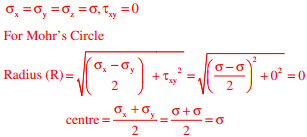Q2. The wastewater form a city, containing a high concentration of biodegradable organics, is being steadily discharged into a flowing river at a location S. If the rate of aeration of the river water is lower than the rate of degradation of the organics, then the dissolved oxygen of the river water
Explaination / Solution:
No Explaination.

Q3. A super-elevation e is provided on a circular horizontal curve such that a vehicle can be stopped on the curve without sliding. Assuming a design speed v and maximum coefficient of side friction fmax, which one of the following criteria should be satisfied?
Explaination / Solution: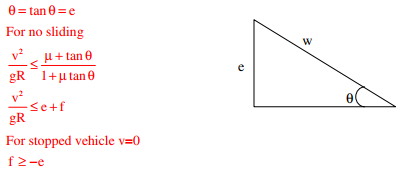Q4. The observed bearings of a traverse are given below:The stations(s) most likely to be affected by the local attraction is/are
Explaination / Solution:So, local attraction at only R

Q5. Consider the following sentences: All benches are beds. No bed is a bulb. Some bulbs are lamps. Which of the following can be inferred? i. Some beds are lamps. ii. Some lamps are beds.
Explaination / Solution:Since there is no direct relation given between lamps and beds. So, neither will be correct

Q6. The function f(x) = 2x - x2 - x3 + 3  has
Explaination / Solution: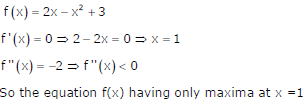Q7. Pre-cursors to photochemical oxidants are
Explaination / Solution:
No Explaination.

Q8. The figure shows a two-hinged parabolic arch of span L subjected to a uniformly distributed load of intensity q per unit length.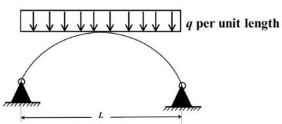The maximum bending moment in the arch is equal to
Explaination / Solution:

Bending moment at any point for two-hinged parabolic arch with uniformly distributed load is zero.

Q9. The accuracy of an Electronic Distance Measuring Instrument (EDMI) is specified as ± (a mm + b ppm). Which one of the following statements is correct?
Explaination / Solution:

Accuracy of EDMI is generally stated in terms of constant instrument error and measuring error proportional to distance being measured. ± (a mm + b ppm) The first part in this expression indicates a constant instrument error that is independent of length of line measured. Second component is distance related error.

Q10.  Group I contains three broad classes of irrigation supply canal outlets. Group II presents hydraulic performance attributes.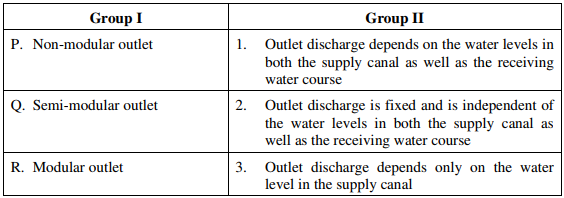The correct match of the items in Group I with the items in Group II is
Explaination / Solution:
No Explaination.